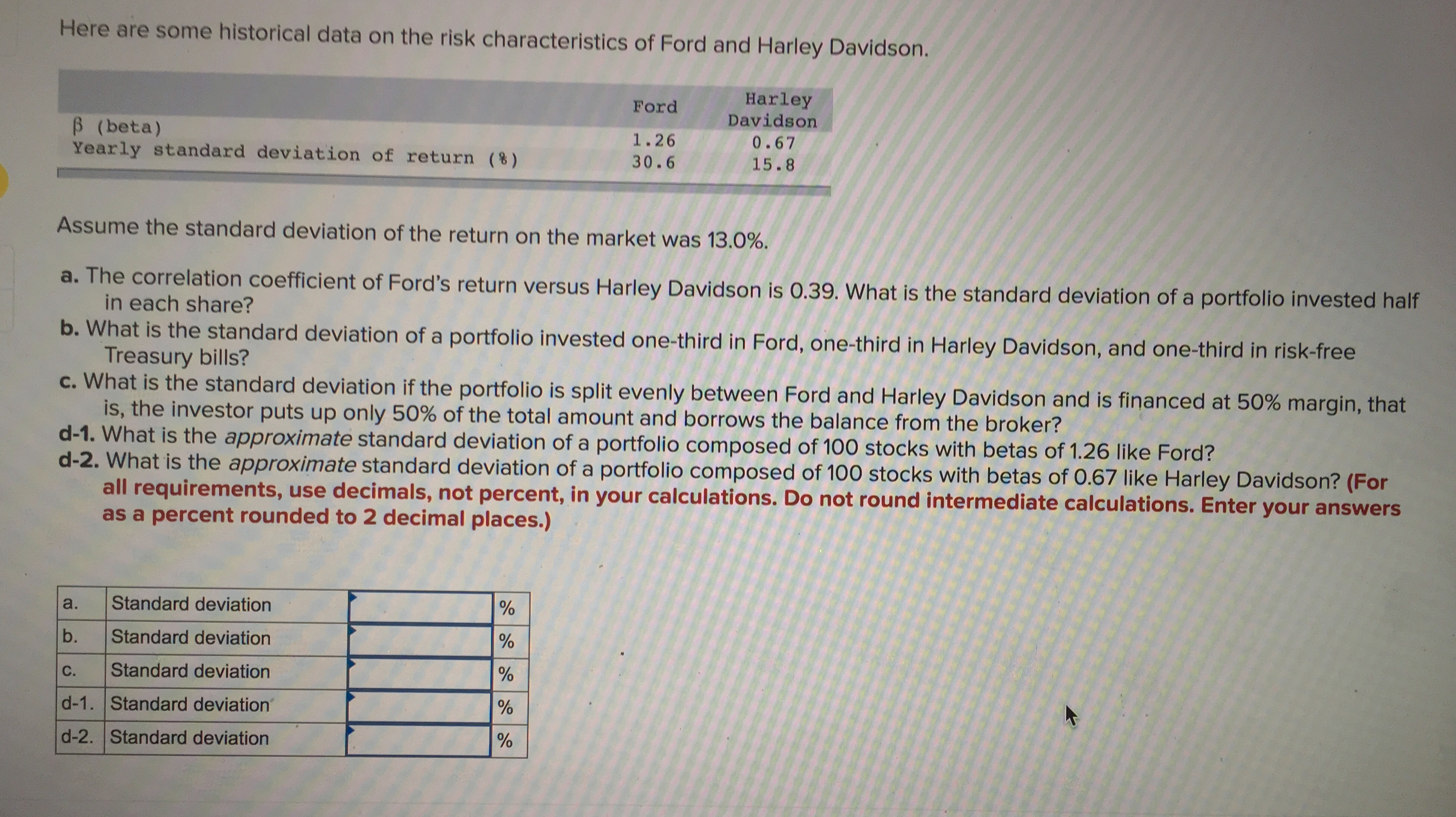# Here are some historical data on the risk characteristics of Ford and Harley Davidson.HarleyDavidsonFordB (beta)Yearly standard deviation of return (%)1.260.6730.615.8Assume the standard deviation of the return on the market was 13.0%a. The correlation coefficient of Ford's return versus Harley Davidson is 0.39. What is the standard deviation of a portfolio invested halfin each share?b. What is the standard deviation of a portfolio invested one-third in Ford, one-third in Harley Davidson, and one-third in risk-freeTreasury bills?c. What is the standard deviation if the portfolio is split evenly between Ford and Harley Davidson and is financed at 50% margin, thatis, the investor puts up only 50% of the total amount and borrows the balance from the broker?d-1. What is the approximate standard deviation of a portfolio composed of 100 stocks with betas of 1.26 like Ford?d-2. What is the approximate standard deviation of a portfolio composed of 100 stocks with betas of 0.67 like Harley Davidson? (Forall requirements, use decimals, not percent, in your calculations. Do not round intermediate calculations. Enter your answersas a percent rounded to 2 decimal places.)Standard deviationa.Standard deviationb.Standard deviationC.d-1. Standard deviationd-2. Standard deviation

Question
243 viewshelp_outlineImage TranscriptioncloseHere are some historical data on the risk characteristics of Ford and Harley Davidson. Harley Davidson Ford B (beta) Yearly standard deviation of return (%) 1.26 0.67 30.6 15.8 Assume the standard deviation of the return on the market was 13.0% a. The correlation coefficient of Ford's return versus Harley Davidson is 0.39. What is the standard deviation of a portfolio invested half in each share? b. What is the standard deviation of a portfolio invested one-third in Ford, one-third in Harley Davidson, and one-third in risk-free Treasury bills? c. What is the standard deviation if the portfolio is split evenly between Ford and Harley Davidson and is financed at 50% margin, that is, the investor puts up only 50% of the total amount and borrows the balance from the broker? d-1. What is the approximate standard deviation of a portfolio composed of 100 stocks with betas of 1.26 like Ford? d-2. What is the approximate standard deviation of a portfolio composed of 100 stocks with betas of 0.67 like Harley Davidson? (For all requirements, use decimals, not percent, in your calculations. Do not round intermediate calculations. Enter your answers as a percent rounded to 2 decimal places.) Standard deviation a. Standard deviation b. Standard deviation C. d-1. Standard deviation d-2. Standard deviation fullscreen
check_circle

Step 1

Since you have asked a question with five sub parts, I will address the first three sub parts. Please post the balance sub parts as separate question.

Part (a)

Proportion invested in Ford, w = 0.5

Proportion invested in Harley Davidson, 1 – w = 1 – 0.5 = 0.5

σ1 = Standard deviation of returns of Ford = 30.6%

σ2 = Standard deviation of returns of Harley Davidson = 15.8%

ρ = Correlation coefficient = 0.39

Step 2

Hence, Variance of the portfolio = σp2 = (w x σ1)2 + (1 – w)2 x σ22 + 2 x ρ x w x (1 – w) σ1 x σ2 = (0.5 x 30.6%)2 + (0.5 x 15.8%)2 + 2 x 0.39 x 0.5 x 0.5 x 30.6% x 15.8% = 0.03907786

Hence, standard deviation of the portfolio = σp = (Variance)1/2 = (0.03907786)1/2 = 19.77%

Step 3

Part (b)

Proportion invested in Ford, w1 = 1/3

Proportion invested in Harley Davidson, w2 = 1/3

The balance 1/3rd of the portfolio is invested in risk free asset that has standard deviation of 0%.

σ1 = Standard deviation of returns of Ford = 30.6%

σ2 = Standard deviation of returns of Harley Davidson = 15.8%

ρ = Correlation coefficient = 0.39

Hence, Variance of the portfolio = σp2 = (w x σ1)2 + (w2 x σ2)2 + 2 x...

### Want to see the full answer?

See Solution

#### Want to see this answer and more?

Solutions are written by subject experts who are available 24/7. Questions are typically answered within 1 hour.*

See Solution
*Response times may vary by subject and question.
Tagged in